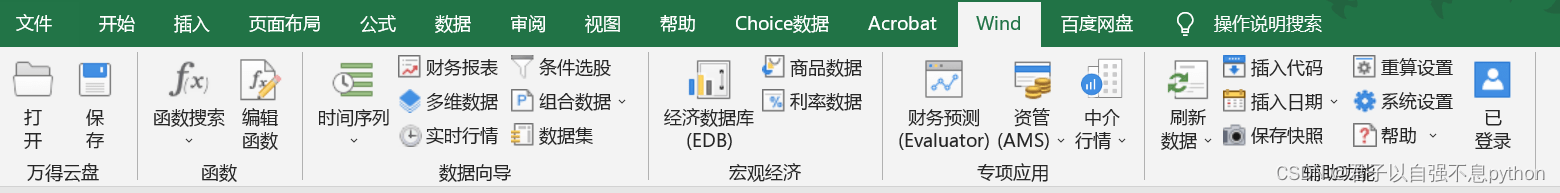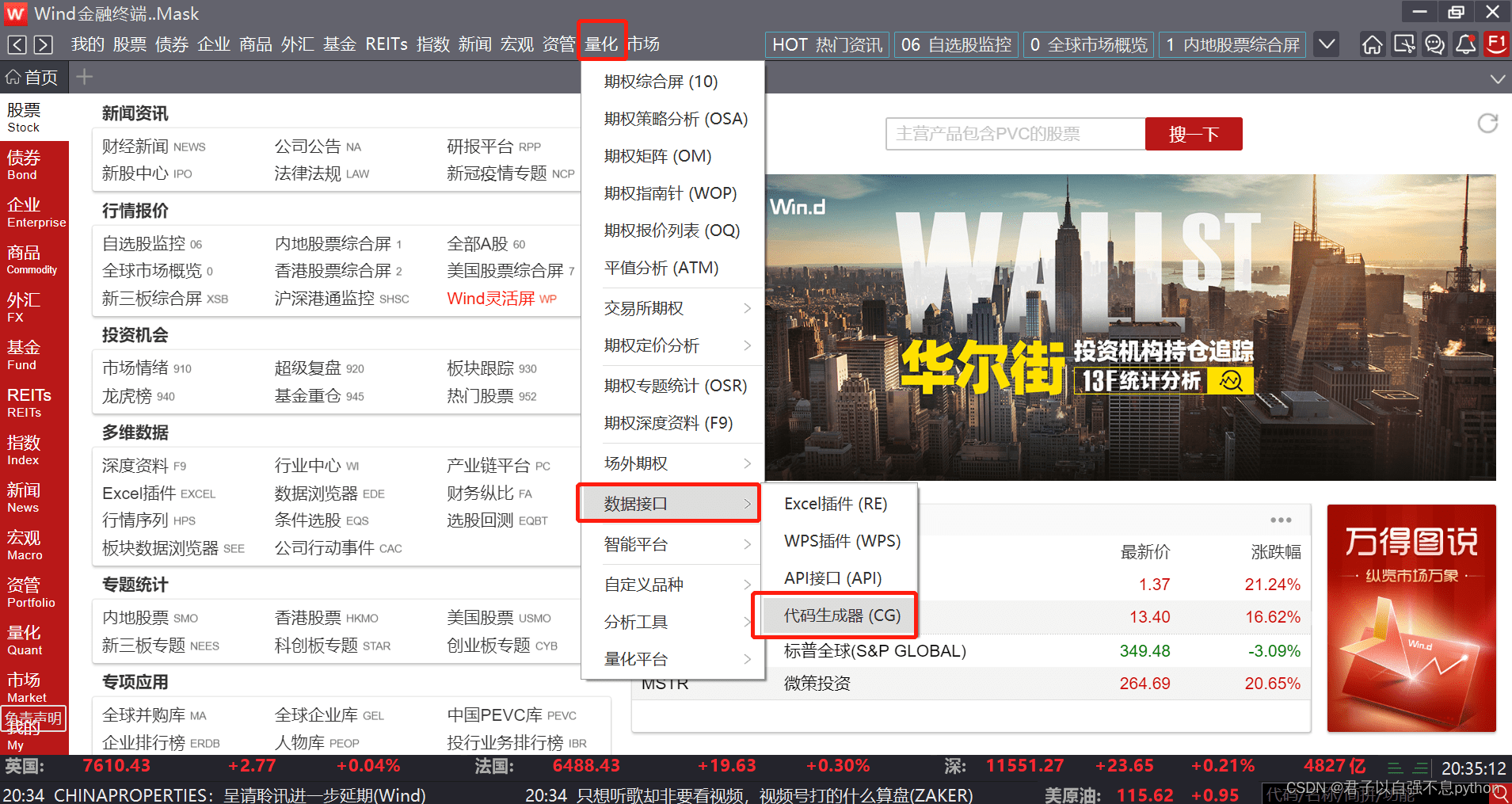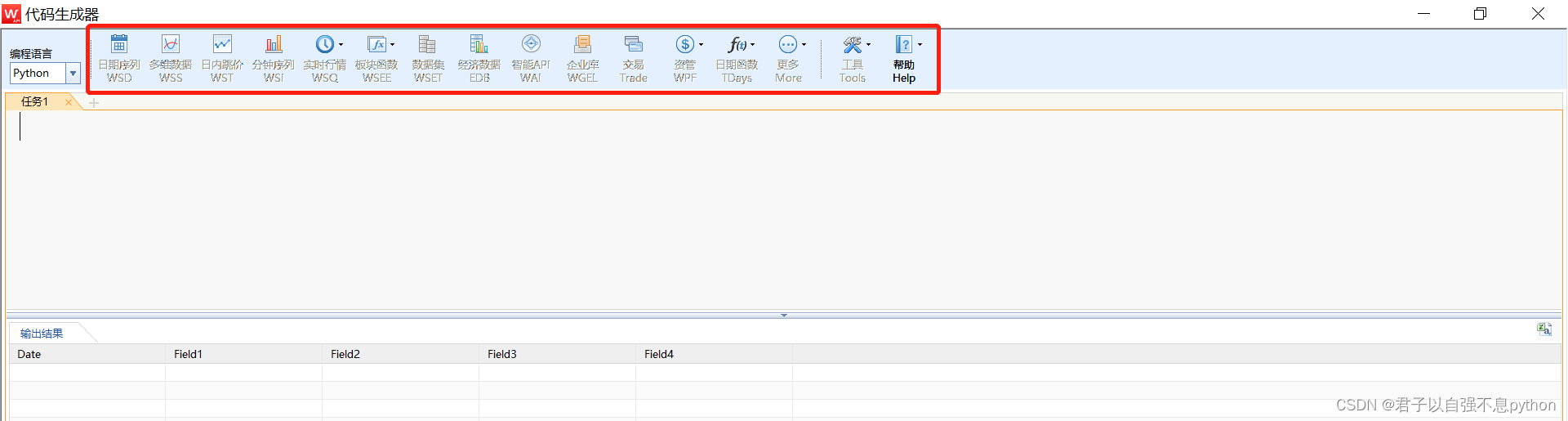# python金融分析小知识(27)——如何通过python连接Wind(万得)数据库

Hello 大家好，我是一名新来的金融领域打工人，日常分享一些python知识，都是自己在学习生活中遇到的一些问题，分享给大家，希望对大家有一定的帮助！1. 修复python接口2.通过python连接Wind数据库

``````## 连接Wind接口
import pandas as pd
from WindPy import w
w.start()``````3.代码生成器的使用``````## 获取融资融券交易金额
data = w.edb("M0075989", "2013-01-04", "2022-05-31","Fill=Previous")

## 获取万得全A(除科创板)成交额 单位元
data2 = w.wsd("881008.WI", "amt", "2013-01-04", "2022-05-30", "")

## 获取上证综指
data3 = w.edb("M0020188", "2013-01-04", "2022-05-31","Fill=Previous")
``````

4.整理获取的数据

``````## 获取融资融券交易金额
data = w.edb("M0075989", "2013-01-04", "2022-05-31","Fill=Previous")
df = pd.DataFrame(data=data.Data,index=data.Times,columns=['融资融券交易金额'])
## 获取万得全A(除科创板)成交额 单位元
data2 = w.wsd("881008.WI", "amt", "2013-01-04", "2022-05-30", "")
df2 = pd.DataFrame(data=data2.Data, index=data2.Times,columns=['万得全A(除科创板)成交额'])
df2 = df2['万得全A(除科创板)成交额'] / 100000000
result = pd.merge(df,df2,left_index=True,right_index=True)
result['融资融券交易金额占比万得全A成交额'] = result['融资融券交易金额'] / result['万得全A(除科创板)成交额']
## 获取上证综指
data3 = w.edb("M0020188", "2013-01-04", "2022-05-31","Fill=Previous")
df3 = pd.DataFrame(data=data3.Data,index=data3.Times,columns=['上证综合指数'])
## 组合数据
result = pd.merge(result,df3,left_index=True,right_index=True)
result``````5.可视化展示

``````## 绘图
import matplotlib.pyplot as plt
%config InlineBackend.figure_format = 'retina'
plt.rcParams['font.sans-serif'] = ['SimHei'] # 指定默认字体
plt.rcParams['axes.unicode_minus'] = False  # 用来正常显示负号
fig, ax = plt.subplots(figsize = (14,6))
ax.plot(result.index, result['融资融券交易金额占比万得全A成交额'], label = '融资融券交易金额占比万得全A成交额', color = 'firebrick')
ax.axhline(y=0.12,linestyle='--',color = 'firebrick', lw = 2.5)
ax.axhline(y=0.06,linestyle='--',color = 'firebrick', lw = 2.5)
ax2 = ax.twinx()
ax2.plot(result['上证综合指数'], label = '上证综合指数', color = 'darkorange')
lines_1, labels_1 = ax.get_legend_handles_labels()
lines_2, labels_2 = ax2.get_legend_handles_labels()
lines = lines_1 + lines_2
labels = labels_1 + labels_2
ax2.legend(lines, labels, loc='best', frameon=False)``````